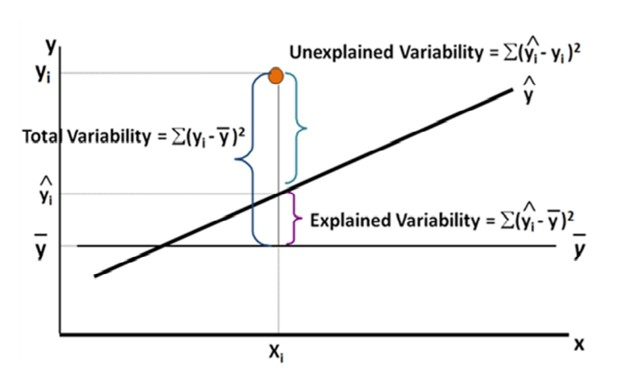Why R2 need to adjust

## Why R-Square need to adjust?Dec 06, 2021

R-square and adjusted R-square values are the two most used parameters for evaluating a regression model. In this discussion we are going to see the difference between both parameters and when to use which, so let’s start with a brief introduction of the parameters:.

R-square

It is an evaluation metric for a regression model defined as the proportion of the variation in the dependent variable explained by the independent variable. The value of R2  determines that out of total change in the dependent variable how much is caused by the independent variable. It is calculated based on the values of total variation in the dependent values and the variation in the predicted values( or sum of residuals).

The value of R2 ranges from 0 to 1 or 0 to 100%, and R2 of 1 means that the total variation in the dependent variable is fully explained by the independent variable. R2 is directly proportional to the correlation between the independent and dependent variable. We can say that higher the correlation, higher the R2 value and better the model will perform. Practically the value of R2 will become zero when the regression model will start performing like the averaging model. We can visualize the regression model with the below figure.The equation of R2 is given as:

R2 =1 – SSR/SST

Where,

SSR = The sum of residual errors(Unexplained variability in fig.)

SST =  The total Variation of the points (Total variability in fig.)

Drawback of R2

The problem with the R2 model is that whenever we add new independent variables in our model the R2 value will increase irrespective of the fact that the added independent variable is improving the model or not. So, by looking at the value of R2 we cannot judge whether the added new feature is improving the model or not.